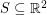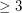# Ulam, Stanislaw M.

## Are there an infinite number of lucky primes? ★

Author(s): Lazarus: Gardiner: Metropolis; Ulam

Conjecture   If every second positive integer except 2 is remaining, then every third remaining integer except 3, then every fourth remaining integer etc. , an infinite number of the remaining integers are prime.

Keywords: lucky; prime; seive

## Dense rational distance sets in the plane ★★★

Author(s): Ulam

Problem   Does there exist a dense setso that all pairwise distances between points inare rational?

Keywords: integral distance; rational distance

## Reconstruction conjecture ★★★★

Author(s): Kelly; Ulam

The deck of a graphis the multiset consisting of all unlabelled subgraphs obtained fromby deleting a vertex in all possible ways (counted according to multiplicity).

Conjecture   If two graphs onvertices have the same deck, then they are isomorphic.

Keywords: reconstruction